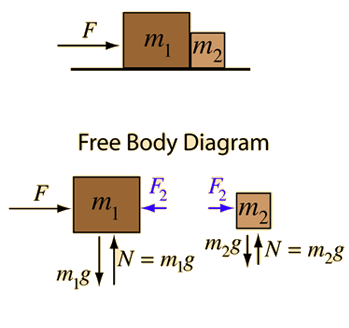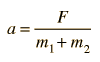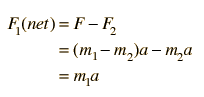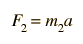# Force on Two MassesSince F is the only net force acting on the two masses, it determines the acceleration of both:The force F2 acting on the smaller mass may now be determined.Note that by Newton's third law, the force F2 acts backward on m1. Note that the net force acting on m1 is consistent with the above.### Calculation

Index

Newton's laws

Standard mechanics problems

 HyperPhysics***** Mechanics R Nave
Go Back

# Force on Two MassesApplication of Newton's second law to two masses.
For a force F= N applied to two masses m1=kg and m2=kg which are in contact with each other, the acceleration is given by= m/s²
Then the force exerted on mass m2 can be determined.= N

### Expressions

Index

Newton's laws

Standard mechanics problems

 HyperPhysics***** Mechanics R Nave
Go Back